#### Vol. 34, No. 2, 1970

 Download this articleFor screen For printingRecent Issues Vol. 325: 1 Vol. 324: 1  2 Vol. 323: 1  2 Vol. 322: 1  2 Vol. 321: 1  2 Vol. 320: 1  2 Vol. 319: 1  2 Vol. 318: 1  2Online Archive Volume: Issue:The Journal Subscriptions Editorial Board Officers Contacts Submission Guidelines Submission Form Policies for Authors ISSN: 1945-5844 (e-only) ISSN: 0030-8730 (print) Special Issues Author Index To Appear Other MSP Journals
Finite transformation formulae involving the Legendre symbol

### Kenneth S. Williams

Vol. 34 (1970), No. 2, 559–568
##### Abstract

Let p denote an odd prime. The following three identities (transformation formulae) involving the Legendre symbol (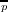) are known to be valid for any complex-valued function F defined on the integers, which is periodic with period p:

 ∑ x=0p−1F(x) + ∑ x=0p−1(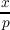)F(x) = ∑ x=0p−1F(x2), ∑ x=0p−1F(x) + ∑ x=0p−1(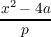)F(x) = ∑ x=1p−1F(x +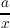),a≢0( mod p), ∑ x=0p−1F(x) + ∑ x=0p−1(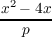)F(x) = ∑ x=1p−1F(x + 2 +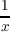).
We consider a general class of transformation formulae, which includes the above examples.

Primary: 10.43
##### Milestones